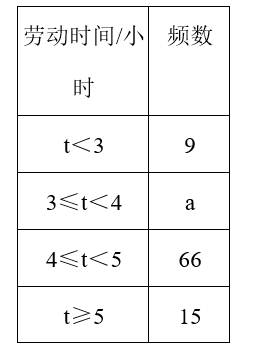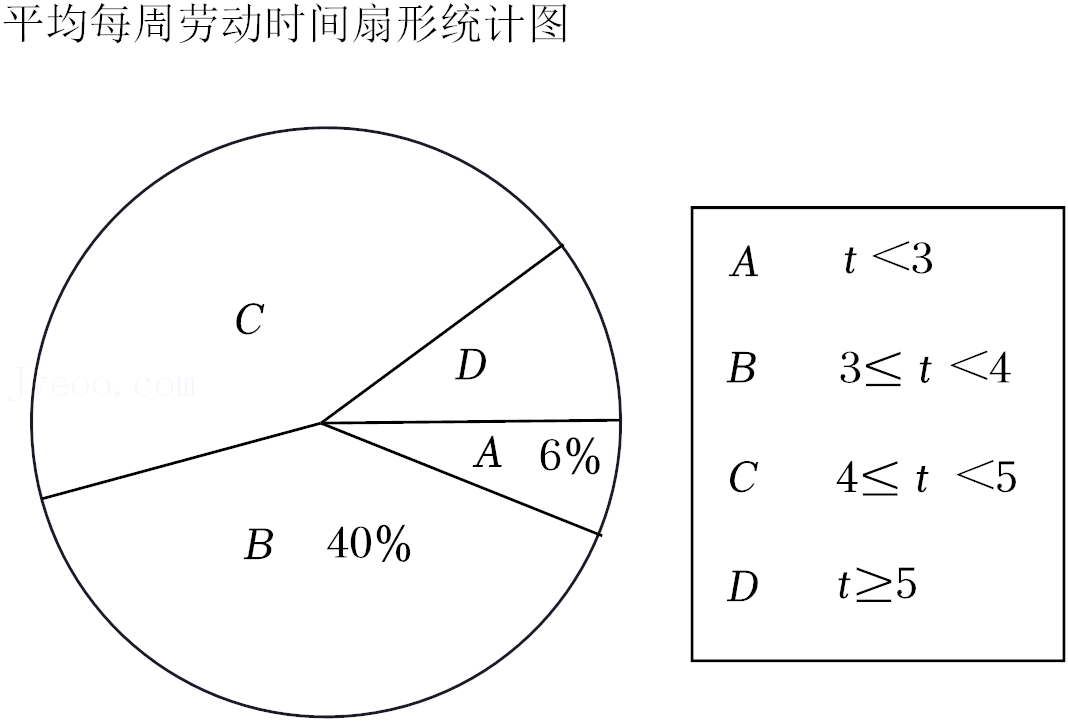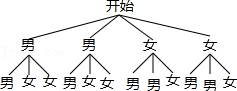（1）参加此次调查的总人数是 （　　） 人，频数统计表中$a＝$
（2）在扇形统计图中，D组所在扇形的圆心角度数是 （　　） ；
（3）该校准备开展以“劳动美”为主题的教育活动，要从报名的2男2女中随机挑选2人在活动中分享劳动心得，请用树状图或列表法求恰好抽到一名男生和一名女生的概率．【答案】 解: (1) 参加此次调查的总人数是: $9 \div 6 \%=150$ (人), 频数统计表中 $\mathrm{a}=150 \times 40 \%=60$,

(2) $\mathrm{D}$ 组所在扇形的圆心角度数是: $360^{\circ} \times \frac{15}{150}=36^{\circ}$,

（3）画树状图如下: